# Plus One Math's Solution miscellaneous Chapter 1 Sets

As per the CBSE norms, Keralanotes expert tutors have prepared NCERT maths solutions for Class 11 Maths Chapter 1 miscellaneous Exercise according to the CBSE Board guidelines. In this chapter, solutions to all questions given in the textbook pertaining to different topics are presented here in an easy manner.

Keralanotes expert tutors have prepared NCERT Solution for Class 11 Maths Chapter 1 miscellaneous Exercise according to the CBSE Board guidelines. The solutions to all questions given in the textbook pertaining to different topics are presented here in an easy manner.

 Board SCERT, Kerala Text Book NCERT Based Class Plus One Subject Math's Textbook Solution Chapter Chapter 1 Exercise Miscellaneous Exercise Chapter Name Sets Category Plus One Kerala

## Kerala Syllabus Plus One Math's Textbook Solution Chapter  1 Sets Miscellaneous Exercise

### Chapter  1  Sets Textbook Solution

Kerala plus One maths NCERT textbooks, we provide complete solutions for the exercise and answers provided at the end of each chapter. We also cover the entire syllabus given by the Board of secondary education, Kerala state.

### Chapter  1  Sets Exercise  Miscellaneous Exercise

Miscellaneous Exercise Class 11 Maths Question 1.
Decide, among the following sets, which sets are subsets of one and another:
A = {x : x ∈R and x satisfy x2 – 8x + 12 = 0}, B = {2, 4, 6}, C = {2, 4, 6, 8,…}, D = {6}.
Solution.
Here A = {x : x ∈ R and x satisfies x2 – 8x + 12 = 0}
= {x : x ∈ R and (x – 6)(x – 2) = 0} = {2, 6}
B = {2, 4, 6}, C = {2, 4, 6, 8,…….} and D = {6}
Now, A ⊂ B, A ⊂ C, B ⊂ C, D ⊂ A, D ⊂ B and D ⊂ C

Miscellaneous Exercise Class 11 Maths Question 2.
In each of the following, determine whether the statement is true or false. If it is true, prove it. If it is false, give an example.
(i) If x ∈ A and A ∈ B,then x ∈ B
(ii) If A ⊂ B and B ∈ C, then A ∈ C
(iii) If A ⊂ B and B ⊂ C, then A ⊂ C
(iv) If A ⊄ B and B ⊄C, then A ⊄ C
(v) If x ∈ A and A ⊄ B, then x ∈ B
(vi) If A ⊂ B and x ∉ B, then x ∉ A
Solution.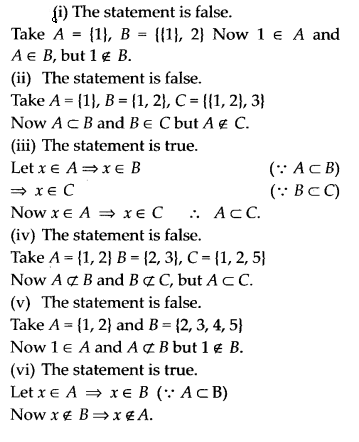Miscellaneous Exercise Class 11 Maths Question 3.
Let A, B and C be the sets such that A ∪ B = A ∪ C and A ∩ B = A ∩ C. Show that B – C.
Solution.
Given that A ∩ B = A ∩C and A ∪ B=A ∪ CMiscellaneous Exercise Class 11 Maths Question 4.
Show that the following four conditions are equivalent :
(i) A ⊂ B
(ii) A – B = φ
(iii) A ∪ B = B
(iv) A ∩ B = A
Solution.
(i) ⇒ (ii)
A – B = {x: x∈ A and x∉B]
Since A⊂B
∴ A – B = φ

(ii) ⇒ (iii)
A – B = φ ⇒ A⊂B ⇒ A∪B = B

(iii) ⇒ (iv)
AuB = B ⇒A⊂B ⇒ A∩B = A

(iv) ⇒ (i)
A∩B = A ⇒ A⊂B
Thus (i) ⇔ (ii) ⇔ (iii) ⇔ (iv).

Miscellaneous Exercise Class 11 Maths Question 5.
Show that if A ⊂ B, then C – B ⊂ C – A.
Solution.
Let x ∈ C – B ⇒x ∈ C and x ∉ B
⇒ x ∈ C and x ∉ A [∵ A ⊂ B]
⇒ x ∈ C – A
Hence C – B ⊂C – A

Miscellaneous Exercise Class 11 Maths Question 6.
Assume that P(A) = P(B). Show that A = B
Solution.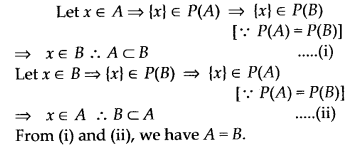Miscellaneous Exercise Class 11 Maths Question 7.
Is it true that for any sets A and B, P(A) ∪ P(B) = P(A ∪ B) Justify your answer.
Solution.
No, it is not true.
Take A = {1, 2} and B = {2,3}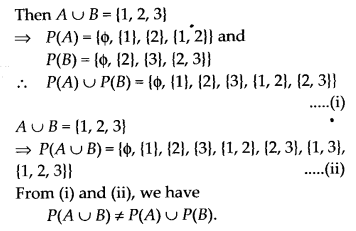Miscellaneous Exercise Class 11 Maths Question 8.
Show that for any sets A and B,
A = (A ∩ B) ∪ (A – B) and A ∪ (B – A) = (A ∪ B)
Solution.
(A ∩ B) ∪ (A – B) = (A ∩ B) ∪ (A ∩ B’)
= A ∩ (B ∪ B’) (By distributive law)
= A ∩ U = A
Hence, A = (A ∩ B) ∪ (A – B)
Also A ∪ (B – A) = A ∪ (B ∩ A’)
= (A ∪ B) ∩ (A ∪ A’) (By distributive law)
= (A ∪ B) ∩ U= A ∪ B
Hence, A ∪ (B – A) = A ∪ B.

Miscellaneous Exercise Class 11 Maths Question 9.
Using properties of sets, show that
(i) A ∪ (A ∩ B)=A
(ii) A ∩ (A ∪ B) = A.
Solution.
(i) We know that if A ⊂ B, then
A ∪ B = B. Also, A ∩ B ⊂ A
∴ A ∪ (A ∩ B) = A.
(ii) We know that if A ⊂ B,
then A ∩ B = A Also, A ⊂ A ∪ B
∴ A ∩ (A ∪ B) = A.

Miscellaneous Exercise Class 11 Maths Question 10.
Show that A ∩ B = A ∩ C need not imply B = C.
Solution.
Let A = {1, 2, 3, 4}, B = {2, 3, 4, 5, 6}, C = {2, 3, 4, 9,10}.
∴ A ∩ B = [1, 2,3,4} ∩ {2,3,4, 5, 6]
= {2, 3, 4}
A ∩ C = {1, 2, 3, 4} ∩ {2, 3, 4, 9, 10} = {2, 3, 4}
Now we have A ∩ B = A ∩ C. But B ≠ C.

Miscellaneous Exercise Class 11 Maths Question 11.
Let A and B be sets. If A ∩ X=B ∩ X = φ and A ∪ X = B  ∪ X for some set X, show that A = B. (Hints A = A ∩ (A∪X), B = B ∩ (B ∪ X) and use Distributive law)
Solution.
Here
A ∪ X = B ∪ X for some set X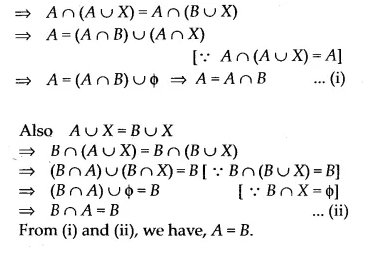Miscellaneous Exercise Class 11 Maths Question 12.
Find sets A, B and C such that A ∩ B, B ∩ C and A ∩ C are non-empty sets and A ∩ B ∩ C = φ
Solution.
Take A = {1, 2}, B = {1, 4} and C = {2, 4}
Now, A ∩ B = {1} ≠ φ, B ∩ C = {4} ≠ φ and
A ∩ C = {2} ≠ φ
But A ∩ B ∩ C = φ.

Miscellaneous Exercise Class 11 Maths Question 13.
In a survey of 600 students in a school, 150 students were found to be taking tea and 225 taking coffee, 100 were taking both tea and coffee. Find how many students were taking neither tea nor coffee?
Solution.
Let T be the set of students who takes tea and C be the set of students who takes coffee. Here, n(T) = 150, n(C) = 225 and n(C ∩ T) = 100
We know that
n(C ∪ T) = n(C) + n(T) – n(C ∩ T)
= 150 + 225 -100 = 275
∴ Number of students taking either tea or coffee = 275
∴ Number of students taking neither tea nor coffee = 600 – 275 = 325.

Miscellaneous Exercise Class 11 Maths Question 14.
In a group of students, 100 students know Hindi, 50 know English and 25 know both. Each of the students knows either Hindi or English. How many students are there in the group?
Solution.
Let H be the set of students who know Hindi and E be the set of students who know English.
Here n(H) = 100, n(E) = 50 and n(H ∩ E) = 25
We know that
n(H ∪ E) = n(H) + n(E) – n(H ∩ E)
= 100 + 50 – 25 = 125

Miscellaneous Exercise Class 11 Maths Question 15.
In a survey of 60 people, it was found that 25 people read newspaper H, 26 read newspaper H, 26 read newspaper I, 9 read both H and I, 11 read both H and T, 8 read both T
and I, 3 read all three newspapers. Find:
(i) the number of people who read at least one of the newspapers.
(ii) the number of people who read exactly one newspaper.
Solution.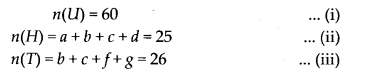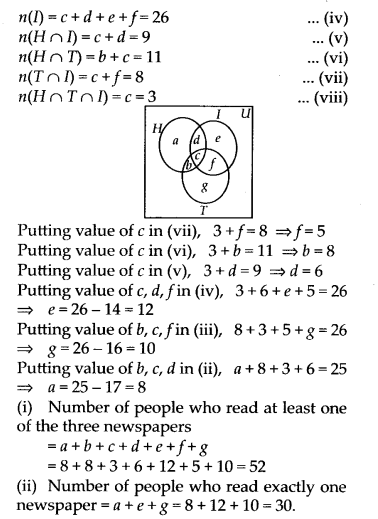Miscellaneous Exercise Class 11 Maths Question 16.
In a survey it was found that 21 people liked product A, 26 liked product B and 29 liked product C. If 14 people liked products A and B, 12 people liked products C and A, 14 people liked products B and C and 8 liked all the three products. Find how many liked product C only.
Solution.#### Chapter 1: Sets Miscellaneous Exercise - Preview

Feel free to comment and share this article if you found it useful. Give your valuable suggestions in the comment session or contact us for any details regarding HSE Kerala Plus one syllabus, Previous year question papers, and other study materials.